### Home > CAAC > Chapter 14 > Lesson 14.2.1.3 > Problem2-39

2-39.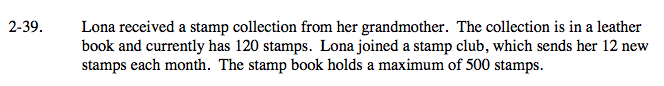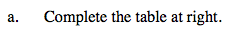Continue adding 12 to find months 2-5.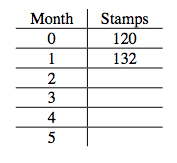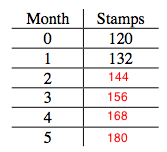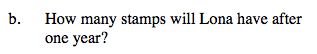See part (a). (Continue until month twelve.)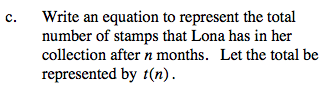Remember that the initial amount was 120 stamps and the rate is 12 stamps per month.

Use these values to make an equation.

t(n) = 120 +12n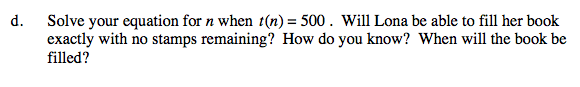Substitute 500 for t(n).

500 = 120 + 12n

Solve for n.

$\textit{n}=31\frac{2}{3}$

Lona will not be able to fill her book exactly, with no leftover stamps, because when the equation is solved for n, the result is a mixed number. It will be filled in 32 months.